# Coordinate Graphing and Distance on Coordinate Planes Lesson Plan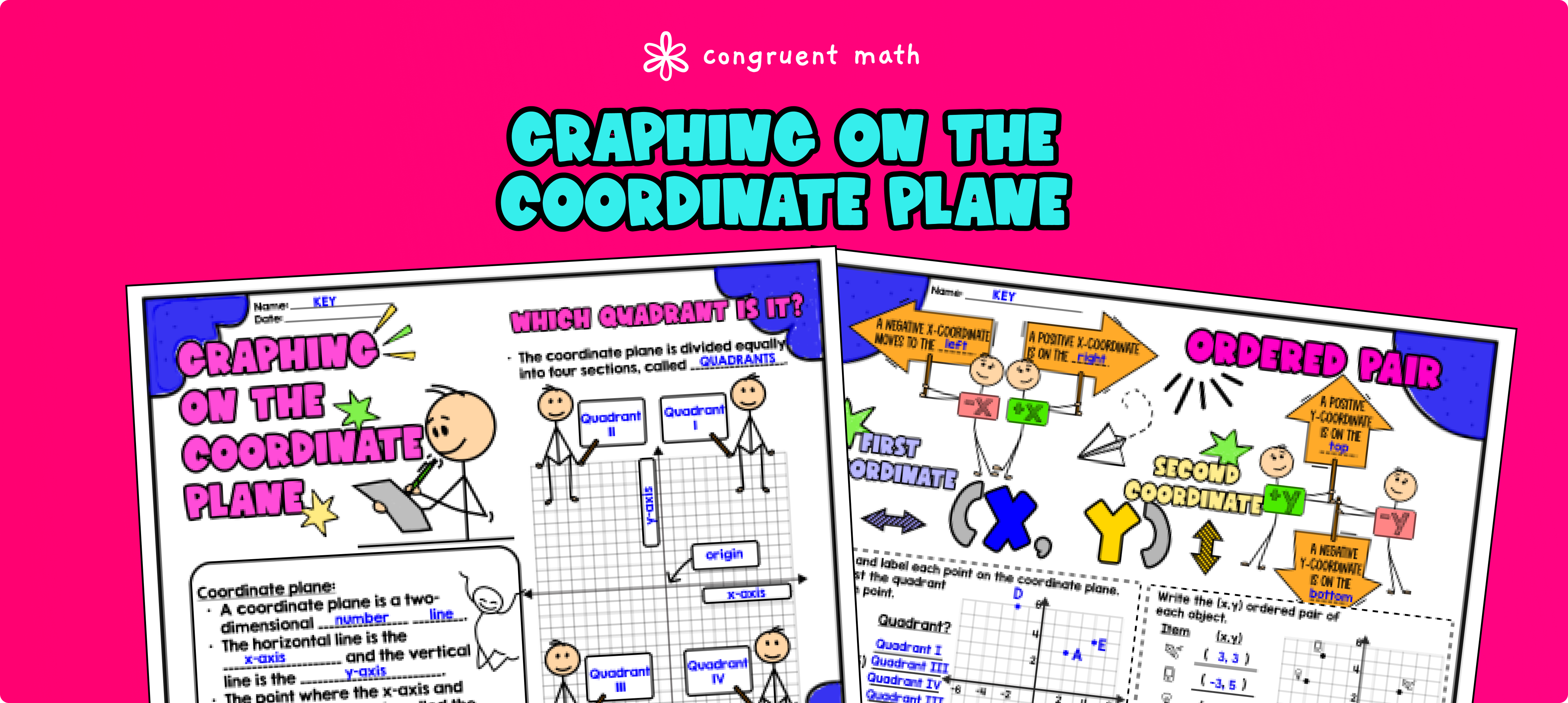Pin This

## Overview

Ever wondered how to teach coordinate graphing and quadrants in an engaging way to your sixth grade students?

In this lesson plan, students will learn about the basics of coordinate planes, quadrants, and how to plot points. Through artistic and interactive guided notes, as well as practice activities such as a doodle and color-by-number activity and a maze worksheet, students will gain a comprehensive understanding of coordinate graphing.

The lesson also covers finding the distance between two points on a coordinate plane, using coordinates to draw polygons, and finding the length of a side joining points with the same first or second coordinate. These concepts are applied to both mathematical and real-world problems.

The lesson culminates with a real-life example that explores how coordinate graphing can be used in real life scenarios.

If you're looking for an introductory lesson on coordinate graphing that is both engaging and comprehensive, this lesson plan is the perfect fit!

## Get the Lesson Materials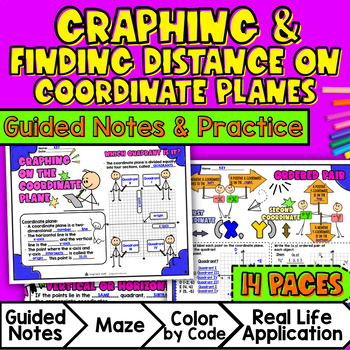\$4.25

## Learning Objectives

After this lesson, students will be able to:

• Identify and define key vocabulary related to coordinate graphing, such as coordinate plane, ordered pairs, and quadrants.

• Plot ordered pairs on all four quadrants of a coordinate plane.

• Calculate the distance between two points on a coordinate plane, specifically when the points share the same first coordinate or the same second coordinate.

• Graph polygons on a coordinate plane using given coordinates for the vertices.

• Use coordinates to find the length of a side joining points with the same first coordinate or the same second coordinate.

• Apply the skills and techniques learned in coordinate graphing to solve real-world and mathematical problems.

## Prerequisites

Before this lesson, students should be familiar with:

• Understanding of the concepts of number lines.

## Key Vocabulary

• Coordinate plane

• Ordered pairs

• x-coordinate, y-coordinate

• Distance

• Polygons

• Vertices

• Area

• Perimeter

## Procedure

### Introduction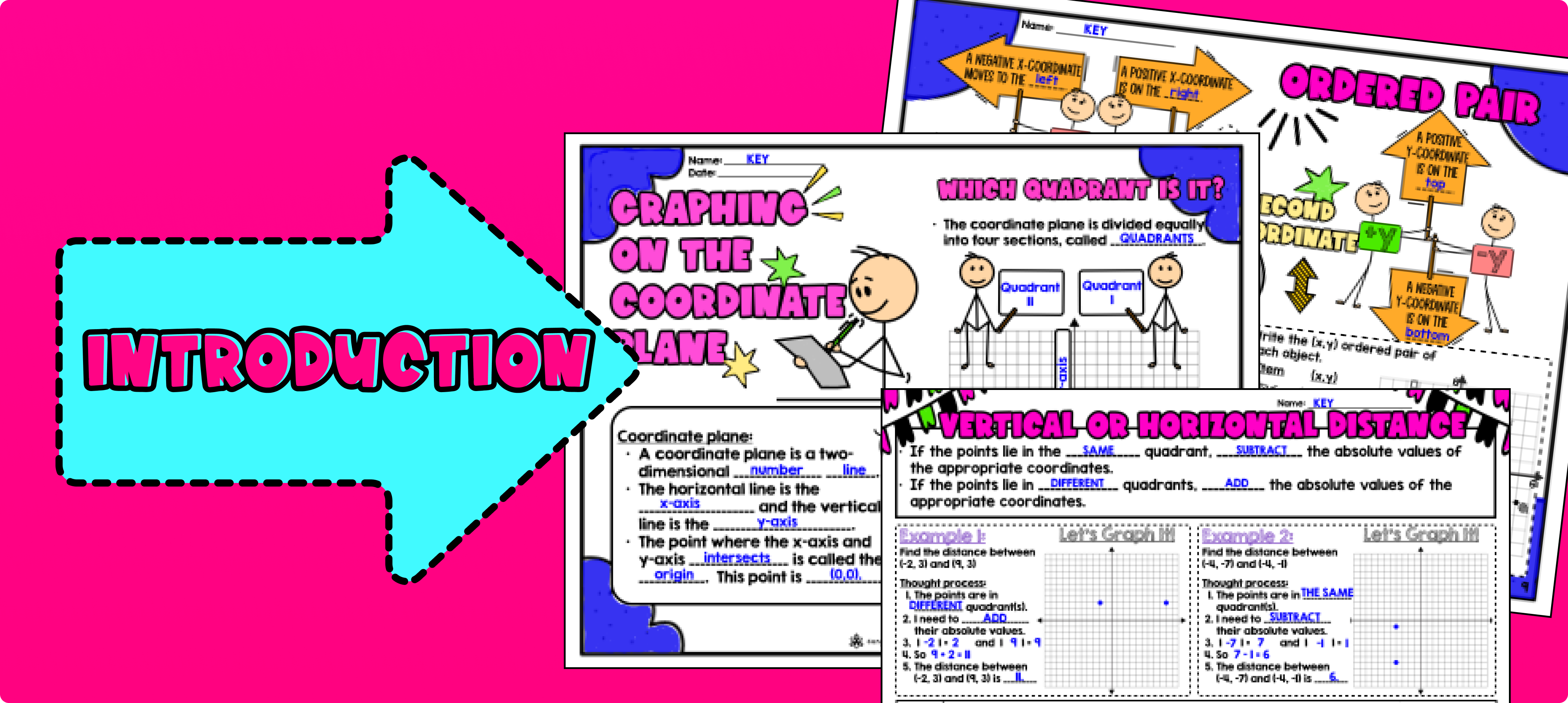Pin This

As a hook, ask students why understanding coordinate planes and graphing points is important in real life. For example, you can ask them how GPS systems or maps use coordinate planes to help them navigate. Refer to the last page of the guided notes as well as the FAQs below for more ideas.

Use the first two pages of the guided notes to introduce the concept of coordinate planes and key vocabulary terms such as coordinate plane, ordered pairs, and quadrants. Walk through the steps of plotting ordered pairs on a coordinate plane. Emphasize the importance of using the x-coordinate and y-coordinate in an ordered pair as horizontal or vertical movement.

Check for Understanding: Have students complete the practice exercises on the first and second page of the guided notes. Call on students to share their answers and explanations. Address any misconceptions or errors that arise during the discussion.

Use the third page of the guided notes to teach students how to find the distance between two points on a coordinate plane. Show them how to use the absolute value of the difference between the x-coordinates or y-coordinates to find the distance as well as graphing on the coordinate plane to find the distance. Provide examples and guide them through the process step by step.

Use the fourth page of the guided notes to explain how to graph polygons on a coordinate plane and find the area and perimeter of each polygon. Give examples of different types of polygons and guide students in identifying the coordinates of the vertices.

Based on student responses, reteach any concepts that students need extra help with. If your class has a wide range of proficiency levels, consider pulling out students for reteaching while more advanced students begin working on the practice exercises.

### Practice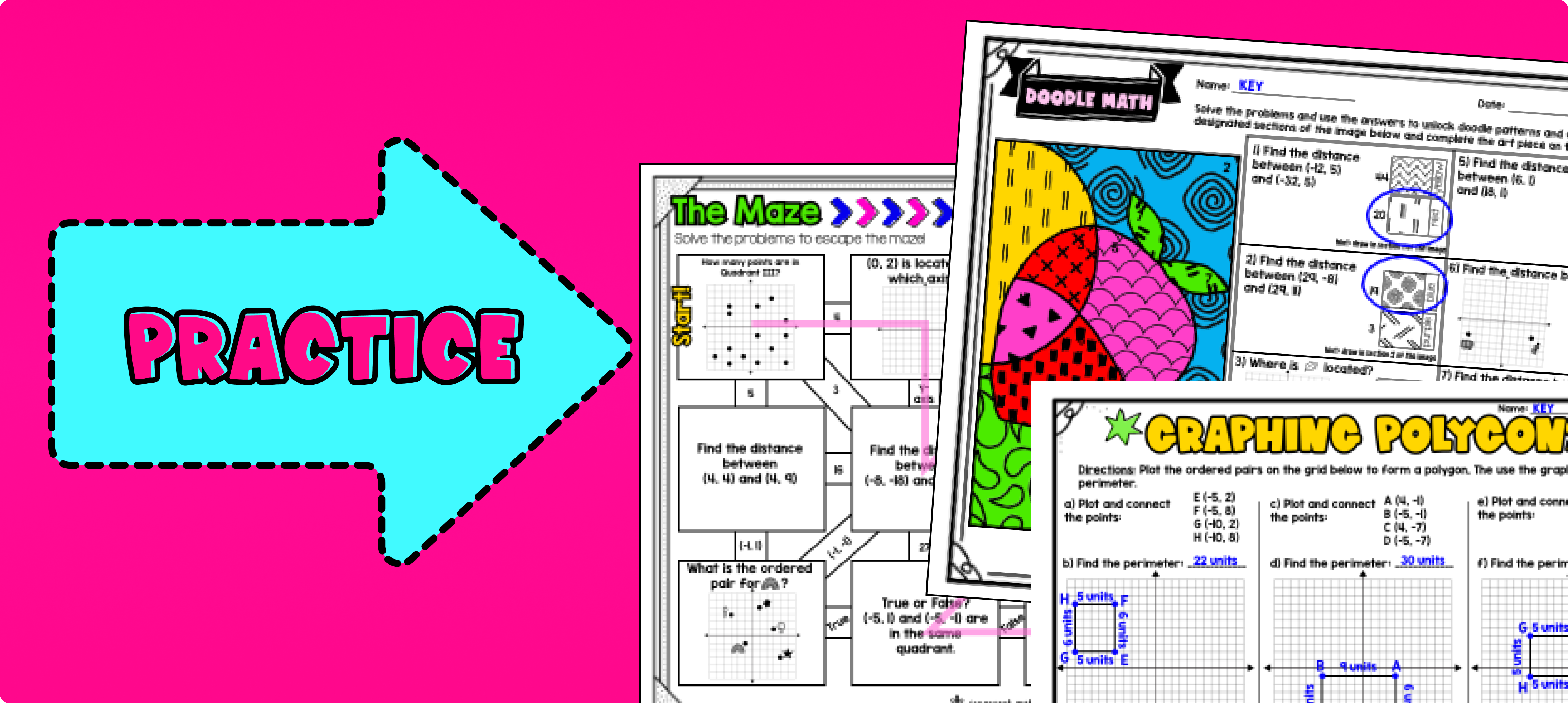Pin This

Have students practice graphing on coordinate planes and finding distances between points using the maze activity Walk around the classroom to answer any questions students may have.

Fast finishers can move on to the doodle math activity for extra practice. You can also assign the doodle math activity as homework for students to complete outside of class.

### Real-Life Application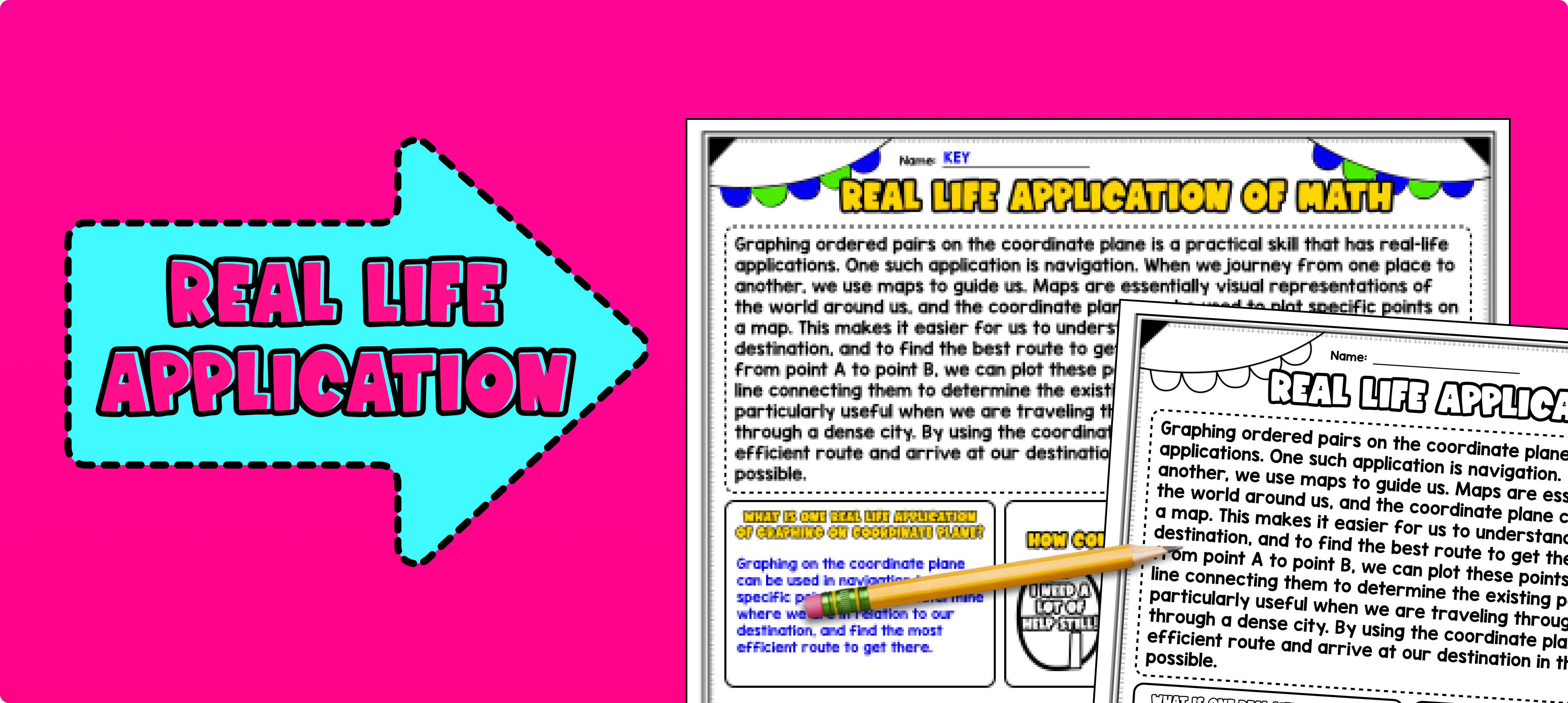Pin This

Bring the class back together, and introduce the concept of using coordinate graphing and finding distances between points in real-life situations. Use the last page of the guided notes resource to explain that coordinate graphing is a useful skill in many fields, including geography, navigation, and urban planning. Allow students to reflect on their learning of this topic by filling in the self assessment section on the real life application page of the guided notes resource.

You can choose to share specific examples related to the interests or experiences of your students. For example, you could discuss how coordinate graphing is used in GPS systems to find the shortest route between two locations. Another example could be explaining how architects and engineers use coordinate graphing to design and construct buildings and structures.

Refer to the FAQ section for more ideas on how to teach real-life applications of coordinate graphing. Encourage students to think about how they can apply this skill in their daily lives or future careers.

## Extensions

If you're looking for digital practice for coordinate graphing and distance on coordinate planes, try these Pixel Art activities in Google Sheets. Every answer is automatically checked, and correct answers unlock parts of a mystery picture. It's incredibly fun and a powerful tool for differentiation.

Here are some activities to explore:

### Real-Life Math Project

A fun way to wrap this lesson with your students is with one of my real-life math projects. It enables students to see the application of the math in an engaging, extended project:

Here is a activity to explore:

## Want more ideas and freebies?

Get my free resource library with digital & print activities—plus tips over email.